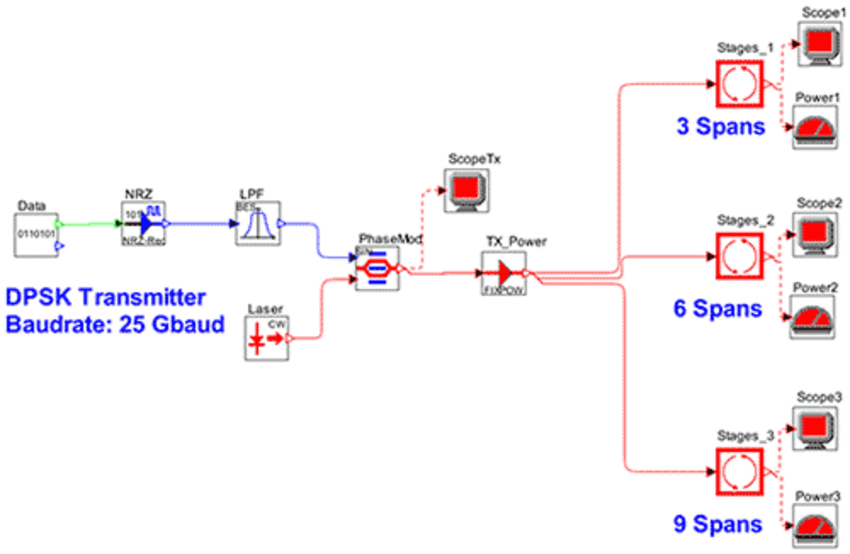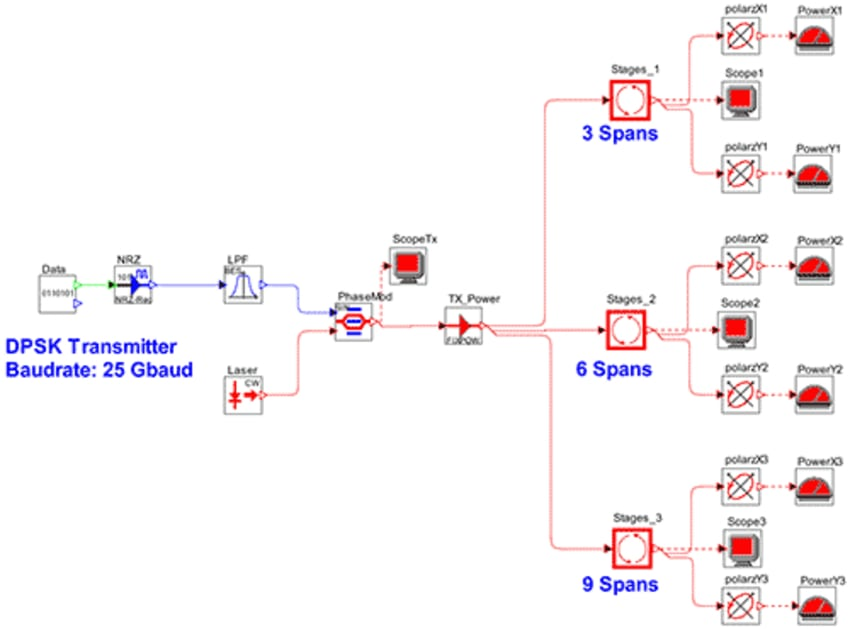# In-Band OSNR Measurement in ROADM-Based Networks Using OptSim

The standard method of measuring optical signal-to-noise ratio (OSNR), as described in the IEC 61280-2-9 test procedure, is based on averaging noise power to the left and right of the channel of interest. This gives what is often referred to as the “out-of-band” OSNR value. In reconfigurable optical add/drop multiplexer (ROADM) based networks, each channel passes through a number of paths, amplifiers and filters. As a result, the noise at the channel wavelength is not the same as the noise to the left and right of the channel. In this case, relying on a standard, out-of-band OSNR measurement can give misleading ideas about network performance. To accurately determine the OSNR, you should calculate the “in-band” OSNR using actual noise levels at the channel wavelength. A number of approaches are suggested in the literature.

This application note describes how OptSim™ users can implement two of the commonly used approaches: (1) independent treatment of signal and noise; and (2) polarization splitting.

Approach 1: Treating Signal and Noise Independently

Figure 1 depicts the schematic layout for a DPSK system used for in-band OSNR measurement. A single-stage ROADM is modeled by a super-Gaussian filter of order 2. Multiple spans represent cascaded stages of amplifiers and ROADMs.  At the end of 3, 6, and 9 spans, optical power meters measure power over a 0.1 nm bandwidth. During the first simulation run, the optical noise is turned off, keeping only the signal of interest. During the second simulation run, the signal of interest is turned off (by setting the “Source Status” parameter in the laser to 0) and only the optical noise is modeled.Figure 1. Simulation setup for in-band OSNR measurement used in the first approach

In other words, the signal power and the noise power are measured independently. The in-band OSNR is calculated by taking the ratio of the two.

Approach 2: Polarization-Splitting Method

Figure 2 shows the schematic layout for a DPSK system used for in-band OSNR measurement based on the polarization-splitting approach [1-2]. The setup and parameters are similar to those used in Approach 1 except for the polarizers used at the outputs of the 3-, 6- and 9-span sections to separate out the polarizations. In each case, the upper polarizer separates out the X polarization, while the lower polarizer separates out the Y polarization. The optical power meters measure power over a 0.1 nm bandwidth for each polarization. The X component is the signal plus half the noise, while the Y component is half the noise and no signal . In other words:

Px in W = Psignal + ½·Pnoise
Py in W = ½·Pnoise
OSNR(dB) = 10·log10[(Px-Py)/(2·Py)]
Alternatively, assuming Psignal >> Pnoise: OSNR(dB)=Px(dBm)-Py(dBm)-3.0Figure 2. Simulation setup for in-band OSNR measurement
based on polarization splitting